Documentation

## Explicit MPC Control of a Single-Input-Single-Output Plant

This example shows how to control a double integrator plant under input saturation in Simulink® using explicit MPC.

For an example that controls a double integrator with an implicit MPC controller, see Control of a Single-Input-Single-Output Plant.

### Define Plant Model

The linear open-loop dynamic model is a double integrator.

```plant = tf(1,[1 0 0]); ```

### Design MPC Controller

Create the controller object with sampling period, prediction and control horizons.

```Ts = 0.1; p = 10; m = 3; mpcobj = mpc(plant, Ts, p, m); ```
```-->The "Weights.ManipulatedVariables" property of "mpc" object is empty. Assuming default 0.00000. -->The "Weights.ManipulatedVariablesRate" property of "mpc" object is empty. Assuming default 0.10000. -->The "Weights.OutputVariables" property of "mpc" object is empty. Assuming default 1.00000. ```

Specify actuator saturation limits as MV constraints.

```mpcobj.MV = struct('Min',-1,'Max',1); ```

### Generate Explicit MPC Controller

Explicit MPC executes the equivalent explicit piecewise affine version of the MPC control law defined by traditional implicit MPC. To generate an Explicit MPC from a traditional MPC, you must specify range for each controller state, reference signal, manipulated variable and measured disturbance so that the multi-parametric quadratic programming problem is solved in the parameter space defined by these ranges.

Use the `generateExplicitRange` function to obtain a range structure where you can specify parameter ranges.

```range = generateExplicitRange(mpcobj); ```
```-->Converting the "Model.Plant" property of "mpc" object to state-space. -->Converting model to discrete time. Assuming no disturbance added to measured output channel #1. -->The "Model.Noise" property of the "mpc" object is empty. Assuming white noise on each measured output channel. ```

MPC controller states include states from plant model, disturbance model and noise model in that order. Setting the range of a state variable is sometimes difficult when the state does not correspond to a physical parameter. In that case, multiple runs of open-loop plant simulation with typical reference and disturbance signals are recommended in order to collect data that reflect the ranges of states.

```range.State.Min(:) = [-10;-10]; range.State.Max(:) = [10;10]; ```

Usually you know the practical range of the reference signals being used at the nominal operating point in the plant. The ranges used to generate an explicit MPC controller must be at least as large as the practical range.

```range.Reference.Min = -2; range.Reference.Max = 2; ```

Specify manipulated variable ranges. If the manipulated variables are constrained, the ranges used to generate the explicit MPC controller must be at least as large as these limits.

```range.ManipulatedVariable.Min = -1.1; range.ManipulatedVariable.Max = 1.1; ```

Use `generateExplicitMPC` command to obtain an explicit MPC controller with the specified parameter ranges.

```mpcobjExplicit = generateExplicitMPC(mpcobj, range) ```
``` Regions found / unexplored: 19/ 0 Explicit MPC Controller --------------------------------------------- Controller sample time: 0.1 (seconds) Polyhedral regions: 19 Number of parameters: 4 Is solution simplified: No State Estimation: Default Kalman gain --------------------------------------------- Type 'mpcobjExplicit.MPC' for the original implicit MPC design. Type 'mpcobjExplicit.Range' for the valid range of parameters. Type 'mpcobjExplicit.OptimizationOptions' for the options used in multi-parametric QP computation. Type 'mpcobjExplicit.PiecewiseAffineSolution' for regions and gain in each solution. ```

Use the `simplify` function with the `'exact'` method to join pairs of regions whose corresponding gains are the same and whose union is a convex set. Doing so can reduce memory footprint of the explicit MPC controller without sacrificing any performance.

```mpcobjExplicitSimplified = simplify(mpcobjExplicit, 'exact') ```
``` Regions to analyze: 15/ 15 Explicit MPC Controller --------------------------------------------- Controller sample time: 0.1 (seconds) Polyhedral regions: 15 Number of parameters: 4 Is solution simplified: Yes State Estimation: Default Kalman gain --------------------------------------------- Type 'mpcobjExplicitSimplified.MPC' for the original implicit MPC design. Type 'mpcobjExplicitSimplified.Range' for the valid range of parameters. Type 'mpcobjExplicitSimplified.OptimizationOptions' for the options used in multi-parametric QP computation. Type 'mpcobjExplicitSimplified.PiecewiseAffineSolution' for regions and gain in each solution. ```

The number of piecewise affine regions has been reduced.

### Plot Piecewise Affine Partition

You can review any 2-D section of the piecewise affine partition defined by the explicit MPC control law.

Use `generatePlotParameters` command to obtain a parameter structure where you can specify which 2-D section to plot afterwards.

```params = generatePlotParameters(mpcobjExplicitSimplified); ```

In this example, you plot the first state variable against the second state variable. All the other parameters must be fixed at a value within their respective ranges.

```params.State.Index = []; params.State.Value = []; ```

Fix other reference signals.

```params.Reference.Index = 1; params.Reference.Value = 0; ```

Fix manipulated variables.

```params.ManipulatedVariable.Index = 1; params.ManipulatedVariable.Value = 0; ```

Use `plotSection` command to plot the 2-D section defined previously.

```plotSection(mpcobjExplicitSimplified, params); axis([-4 4 -4 4]); grid xlabel('State #1'); ylabel('State #2'); ```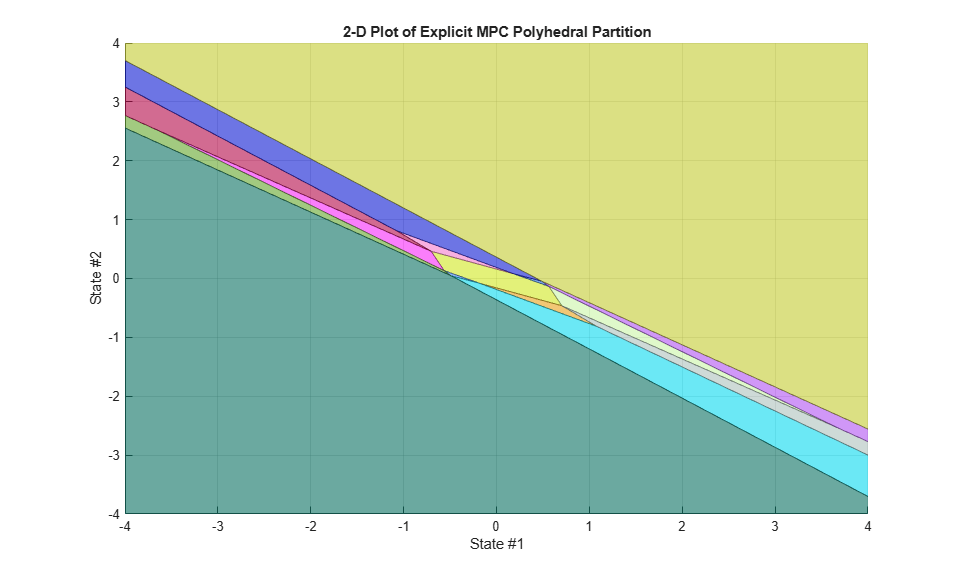### Simulate Using `mpcmove` Function

Compare closed-loop simulations for traditional implicit MPC and explicit MPC using the `mpcmove` and `mpcmoveExplicit` functions respectively.

Prepare to store the closed-loop MPC responses.

```Tf = round(5/Ts); YY = zeros(Tf,1); YYExplicit = zeros(Tf,1); UU = zeros(Tf,1); UUExplicit = zeros(Tf,1); ```

Prepare the real plant used in simulation

```sys = c2d(ss(plant),Ts); xsys = [0;0]; xsysExplicit = xsys; ```

Use an `mpcstate` object to specify the initial states for both controllers.

```xmpc = mpcstate(mpcobj); xmpcExplicit = mpcstate(mpcobjExplicitSimplified); ```

Iteratively simulate the closed-loop response for both controllers.

```for t = 0:Tf % update plant measurement ysys = sys.C*xsys; ysysExplicit = sys.C*xsysExplicit; % compute traditional MPC action u = mpcmove(mpcobj,xmpc,ysys,1); % compute Explicit MPC action uExplicit = mpcmoveExplicit(mpcobjExplicit,xmpcExplicit,ysysExplicit,1); % store signals YY(t+1)=ysys; YYExplicit(t+1)=ysysExplicit; UU(t+1)=u; UUExplicit(t+1)=uExplicit; % update plant state xsys = sys.A*xsys + sys.B*u; xsysExplicit = sys.A*xsysExplicit + sys.B*uExplicit; end fprintf('\nDifference between traditional and Explicit MPC responses using MPCMOVE command is %g\n',... norm(UU-UUExplicit)+norm(YY-YYExplicit)); ```
```Difference between traditional and Explicit MPC responses using MPCMOVE command is 1.88426e-13 ```

### Simulate Using `sim` Function

Compare closed-loop simulation between traditional MPC and Explicit MPC using `sim` commands respectively.

```Tf = 5/Ts; % simulation iterations [y1,t1,u1] = sim(mpcobj,Tf,1); % simulation with tradition MPC [y2,t2,u2] = sim(mpcobjExplicitSimplified,Tf,1); % simulation with Explicit MPC ```
```-->Converting the "Model.Plant" property of "mpc" object to state-space. -->Converting model to discrete time. Assuming no disturbance added to measured output channel #1. -->The "Model.Noise" property of the "mpc" object is empty. Assuming white noise on each measured output channel. ```

The simulation results are identical.

```fprintf('\nDifference between traditional and Explicit MPC responses using SIM command is %g\n',... norm(u2-u1)+norm(y2-y1)); ```
```Difference between traditional and Explicit MPC responses using SIM command is 1.88386e-13 ```

To run this example, Simulink is required.

```if ~mpcchecktoolboxinstalled('simulink') disp('Simulink is required to run this example.') return end ```

Simulate the traditional MPC controller in Simulink. The MPC Controller block is configured to use `mpcobj` as its controller.

```mdl = 'mpc_doubleint'; open_system(mdl) sim(mdl) ```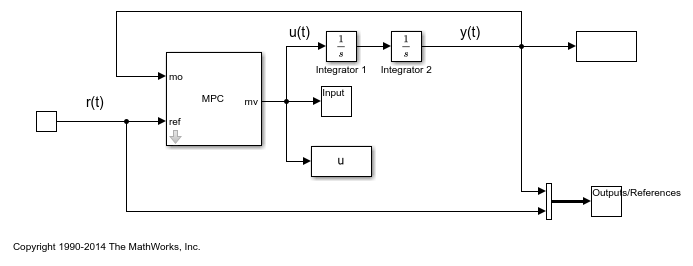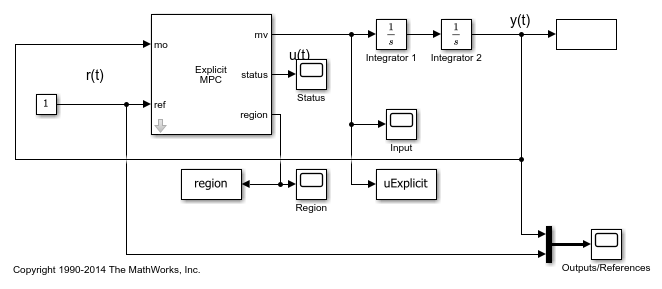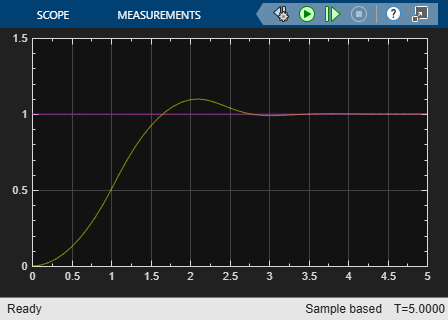Simulate the explicit MPC controller in Simulink. The Explicit MPC Controller block is configured to use `mpcobjExplicitSimplified` as its controller.

```mdlExplicit = 'empc_doubleint'; open_system(mdlExplicit) sim(mdlExplicit) ```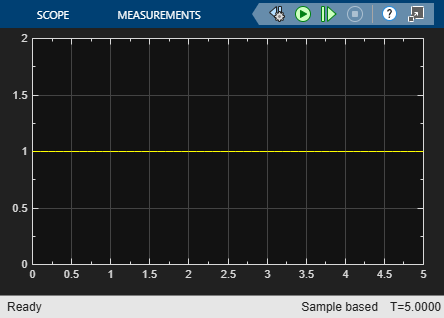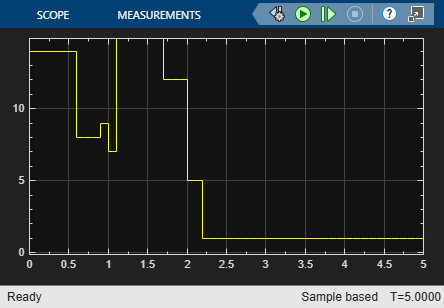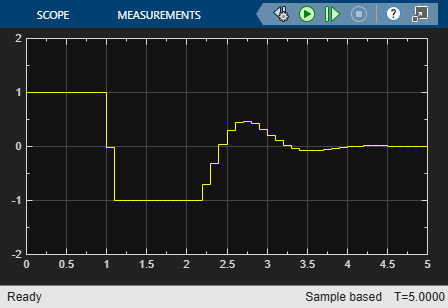The closed-loop responses are identical.

```fprintf('\nDifference between traditional and Explicit MPC responses in Simulink is %g\n',... norm(uExplicit-u)+norm(yExplicit-y)); ```
```Difference between traditional and Explicit MPC responses in Simulink is 1.89531e-13 ```
```bdclose(mdl) bdclose(mdlExplicit) ```